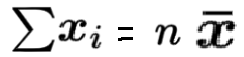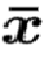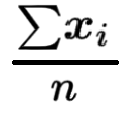Unicminds# Deriving the Formula of Variance without using the Sample Mean

Variance is a measure of how the data points vary from the mean. Since we want the absolute variance, we need to square the distance from the mean on both lower and upper sides. Therefore, the formula of variance is as below.

Below are the steps to deriving the formula of sample variance without using the mean value.

Step 1: Expand the numerator using (a-b)^2

Step 2: Replaceto get the formula with the mean

Step 3: Replace meanwithHope this is useful, thank you.

BOOK A FREE TRIAL